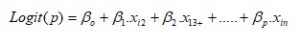# How to apply logistic regression in a case?

By Priya Chetty on December 4, 2017

Machine learning involves solutions to predict scenarios based on past data. Logistic regression offers probability functions based on inputs and their corresponding output. In short, the dependent variable is a classification variable.

One of the most common methods of data analysis is the linear or multiple regression analysis. It helps establish the relationship between independent and dependent variables. In linear regression the dependent variable is continuous in most cases. However,  if the dependent variable is categorical then simple regression will not allow for prediction. So, statisticians introduced the logistic regression method where the dependent variable is non-continuous or it is categorical. Like all regression, it is also used in predictive analysis. However, it helps describe the behavior of a variable which is binary, i.e., which can take only two values. If there are more than two categories in the dependent variable, then multinomial logistic regression is applicable instead of simple logistic regression. Below is an example of how this test works.

## Application of logistic regression

This section contains a case study to explain the application of logistic regression on a dataset. The case dataset here contains two series, series X and Y. Series X indicates the CGPA (Cumulative Grade Points Average) of ten students of a school. Series Y indicates whether these students gets admission in college, where 1= admit, and 0=no admit. The table below represents their values.

 (CGPA) X (Admission)Y 6 0 4 0 7.7 1 10 1 8.9 1 5.5 0 6.7 0 8.9 1 7.6 1 8.7 1

Table 1: Values for logistic regression case 1

Here, logistic regression will help assess what level of CGPA leads to admission in college. This is called a ‘binary classification’ (either 1 or 0) problem. These types of cases need logistic regression. The aim here is to come up with the probability function that takes input X (CGPA) with outcome ‘what is the probability of getting admission’. Hence this test estimates a probability of each student based on its score. Furthermore, based on that probability, the analysis predicts if the student will get admission or not.

Logistic regression analysis is used to get the outcome for following expressions:This function is also called the ‘log of odds’ of an event. On the basis of the above log of odds function the multiple regression equation for logistic regression can be:

##Logistic regression case

Undertaken again a case study of students appearing for job interview, the aim is to determine the applicability of scores of interviews, CGPA, written and aptitude tests in hiring.

The above data was processed using SPSS. The images below show the results of the test on the above data. The first table displays the model summary and the significance level. They indicate if the undertaken variables were enough to ascertain the job status.a. Estimation terminated at iteration number 5 because parameter estimates changed by less than .001. Image 2: Model summary from the logistic regression.

Here, Cox & Snell R squared and Nagelkerke R squared are similar to the R squared in the normal linear regression analysis. This explains the variation in the dependent variable due to independent variables included in the logistic model.

The second table list outs the SPSS generated ‘predicted’ job status, based on the trends in undertaken variables.